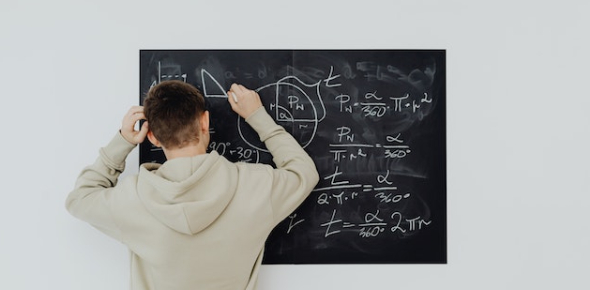# Algebra: Take The Linear Equation Test Quiz!

10 Questions | Attempts: 775SettingsCan you solve the basic linear expression questions? Take the linear equation test quiz and test your knowledge. Did you know that width is the measurement or extent of something from side to side? Were you aware that width is the horizontal measurement that is taken at right angles to the length? Did you know that length is a measure of distance? Have you ever wanted to know how to solve complicated math problems? Look no further than this quiz to start!

• 1.
Willie rented a bike from Beach Bikes. It costs \$18 plus \$5 for each hour. If Willie paid \$48 when he returned his bike, how many hours did he rent the bike?Let x = the hours he rented the bike.
• A.

18x + 5 = 48

• B.

48 - 5 = 18x

• C.

18 + 5x = 48

• D.

None of these

• 2.
Jason received 66 dollars for his birthday.  He went to a sporting goods store and bought a baseball glove, baseball, and bat.  He had 20 dollars left over.  How much did he spend on the baseball gear?  Let g = the amount he spent on the gear.
• A.

66 + 20 = g

• B.

G + 66 = 20

• C.

G + 20 = 66

• D.

None of these

• 3.
The local dog groomer charges \$18 for a dog bath and \$3 for each special service.  If Jonah's total bill was \$27, how many special services were included?  Let x = # of special services
• A.

18 - 27 = 3x

• B.

27 = 18 + 3x

• C.

27 + 3x = 18

• D.

None of these

• 4.
How many ink cartridges can you buy for 105 dollars if one cartridge costs 15 dollars? Let c= the number of ink cartridges you can buy.
• A.

15 - 105 = c

• B.

105 = c - 15

• C.

15 = 105 + c

• D.

None of these

• 5.
There were seven roses in the vase. Sara cut some more roses from her flower garden. There are now 16 roses in the vase. How many roses did she cut? Let r = # of roses she cut.
• A.

R + 7 = 16

• B.

R - 7 = 16

• C.

7r = 16

• D.

None of these

• 6.
Write in slope intercept form: 5x - 3y = -9.
• A.

Y = (5/3)x + 3

• B.

Y = (5/3)x + 9

• C.

Y = (5/3)x - 3

• D.

Y = (3/5)x + 3

• 7.
Solve: 5(y - 1) = 3(2y - 5) - (1 - 3y)
• A.

1/2

• B.

8/12

• C.

23/11

• D.

11/4

• 8.
Solve: (3y - 2)/3 + (2y + 3)/3 = (y + 7)/6
• A.

4/7

• B.

5/9

• C.

2/3

• D.

6/25

• 9.
Solve: (2x - 5)/(3x - 1) = (2x - 1)/(3x + 2)
• A.

-11/6

• B.

6/11

• C.

9/7

• D.

16/5

• 10.
Solve: (0.5y - 9)/0.25 = 4y - 3
• A.

-5.4

• B.

-7.8

• C.

-16.5

• D.

-18.2

## Related TopicsBack to top
×

Wait!
Here's an interesting quiz for you.